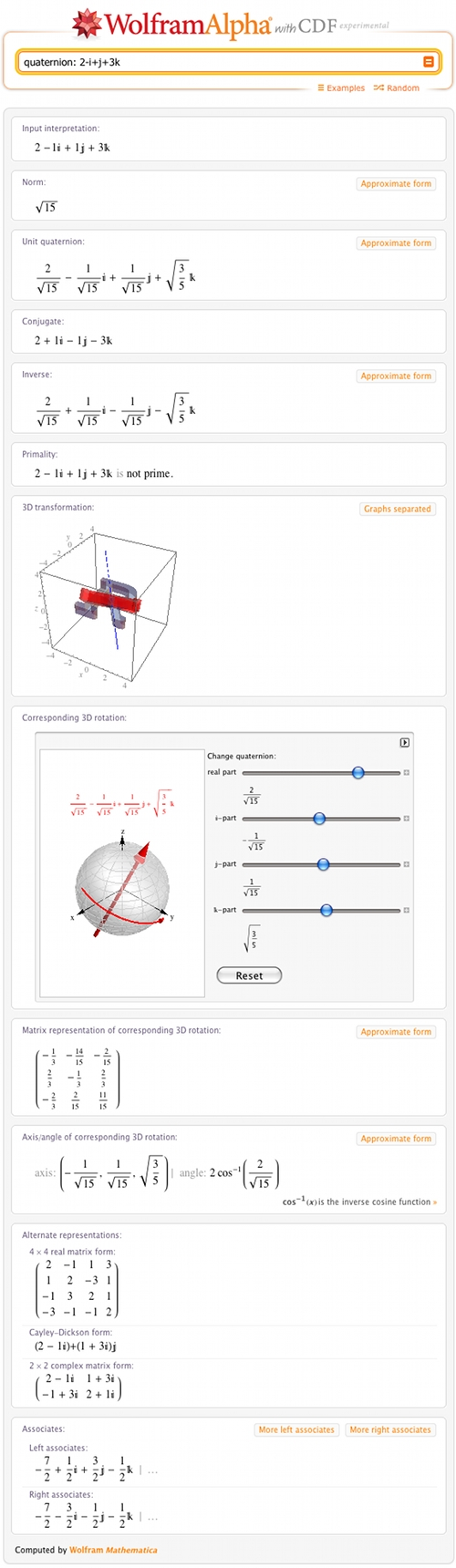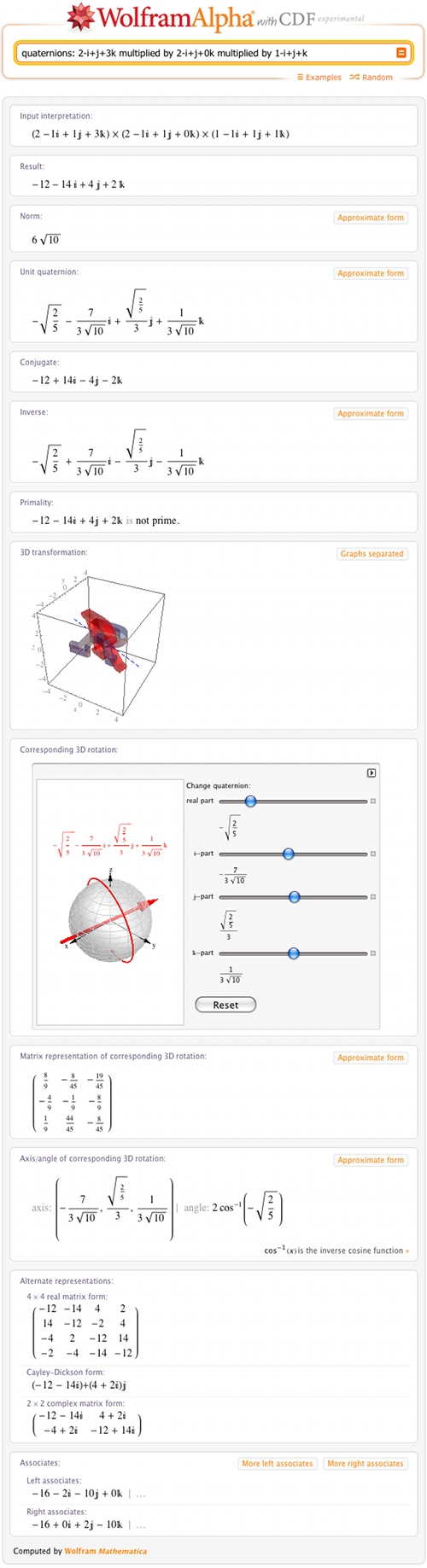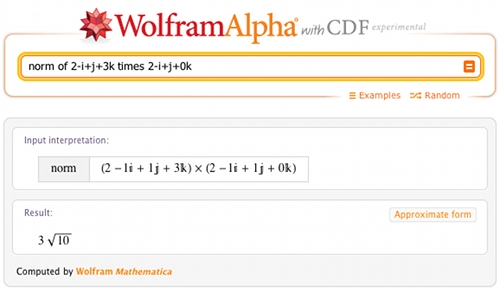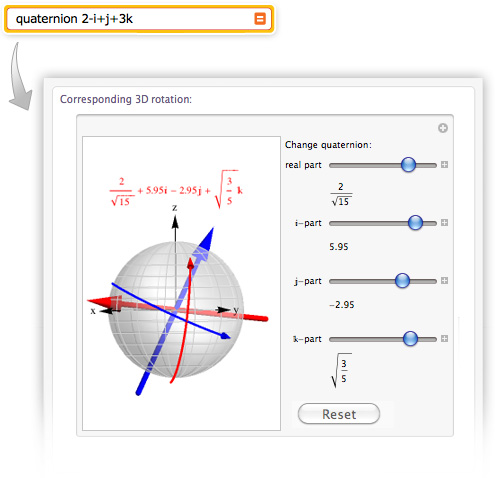# Quaternion Properties and Interactive Rotations with Wolfram|Alpha

August 25, 2011 —Comments Off

One fall evening in 1843, a man walked past the Brougham Bridge along the Royal Canal in Dublin, Ireland. Suddenly, he felt a flash of insight so strong he was compelled to etch his thoughts into the rock on the side of the bridge. This is what he wrote:

i2 = j2 = k2 = i j k = -1

The man was mathematician William Rowan Hamilton, and the insight was of a number system that could represent forces and motions in three-dimensional space. Hamilton called his numbers “quaternions”, because each has four parts: a real number part, and three other parts labeled with i, j, and k, each of which is also a real number. For example, 2 + 3i + 0.342j – 2k is a quaternion.

Quaternions are a generalization of the complex numbers, just like the complex numbers are a generalization of the real numbers. Also like complex numbers, each quaternion has a “norm” (length) and a “conjugate”. Typing a quaternion into Wolfram|Alpha will bring up all of this information and more:Note that this same quaternion can also be entered in the Mathematica form: Quaternion[2,-1,1,3].

Quaternion addition is the same as vector addition—just add the corresponding parts. Quaternion multiplication is very different. For one thing, it’s not commutative: q x r is not always the same as r x q. Wolfram|Alpha will multiply any number of quaternions together and tell you about the result.If you don’t want all of this information, you can ask Wolfram|Alpha for a specific piece information about a given quaternion or product of quaternions:Quaternions are useful as a way of describing many natural phenomena, including Newtonian mechanics, as was Hamilton’s intention. Today, quaternions are mainly used to compute three-dimensional rotations for computer graphics. Wolfram|Alpha provides several representations of the corresponding rotation:By playing with the interactive version of the “Corresponding 3D rotation” pod, you can discover how the four values in a quaternion determine the corresponding rotation. The pod is initially set to the four values of the corresponding unit quaternion and it lets you vary these values from -10 to 10. Since the rotation given by a quaternion is determined only by its corresponding unit quaternion, every possible rotation can be explored.

We hope you find this new feature fun and useful. Enjoy!